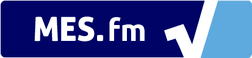We notice that you are using AdBlock. Please whitelist Mortgage Calculator to help keep this site up and running! :)# Mortgage Amortization Formula (Basic)

A = P\frac{i(1+i)^n}{(1+i)^n - 1}

where:

• A = periodic payment amount
• P = amount of principle or loan owing (subtracting any down-payments)
• i = period interest rate
• NOTE: if installments are monthly and interest rate is annual, need to divide by 12
• n = total number of payments

The proof of the formula can be found here: